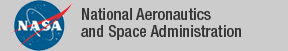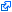### Top File of PVS reals Library

```
top: THEORY
%------------------------------------------------------------------------
% Elementary properties of reals and operations over reals
%
%  top_sigma      -- provides a family of summation functions
%                    over functions [T FROM int -> real]
%  min_max        -- properties on min,max
%  abs_lems       -- additional properties of abs
%  product_real   -- defines product over functions [int -> real]
%  bounded_reals  -- defines sup, inf, max, min
%  real_sets      -- properties of sup, inf, max, min
%  real_fun_ops,  -- adding, subtracting, etc on functions
%  real_fun_preds -- increasing?, decreasing?, etc on functions
%  real_fun_props -- properties about defs in real_fun_preds
%  exponent_props -- additional properties of expt
%  sqrt           -- properties of square root
%  sqrt_rew       -- REVISED with AUTO_REWRITE+ statements
%  sqrt_approx    -- definitions of sqrt_newton and sqrt_bisect
%                    (newton and bisection methods for computing square roots).
%  sq             -- square function and properties
%  sq_rew         -- installs useful AUTO_REWRITE+s
%  sign           -- properties of sign function
%  sign3          -- properties of sign3 function
%  binomial,      -- binomial coefficient
%  polynomials	  -- polynomials
%  more_polynomial_props
%
%  Version 2.5   1/24/08
%
%  CHANGES:
%
%     8/1/01:
%        sqrt_exists    -- existence proof of sqrt
%        sqrt_approx    -- theory added (by Fleuriot/Geser)
%
%     8/7/01
%        sigma_with, sigma_zero added to sigma
%
%     2/26/02
%        duplications with PVS 2.4 prelude removed
%        predicates over functions given names with ?, e.g. increasing?
%
%
%     2/26/03: Added AUTO_REWRITE+ commands to automate some routine stuff
%
%
%     4/14/03: Duplicate theory problem resolved
%
%
%
%     2/13/04: turned on more auto-rewrites in sq and sqrt
%              (if old proofs fail, add the following to your old proof:
%        (STOP-REWRITE "sq_sqrt" "sq_0" "sq_1" "sq_abs" "sq_abs_neg")
%        (STOP-REWRITE "sqrt_0" "sqrt_1" "sqrt_square" "sqrt_sq" "sqrt_sq_neg")
%     7/8/04:  Added auto-rewrites in factorial
%     3/28/05: Added log_nat and moved bolzano.pvs to Interval package
%              by C. Munoz
%     4/15/05: polynomials moved here from trig_fnd
%     3/27/06: sigma theories generalized by Paul Miner
%     11/7/07: sigma theories improved
%     1/24/08: product operator theories added
%
%------------------------------------------------------------------------

BEGIN

IMPORTING top_sigma,         % set of summation theories
mixed_products,
mixed_sigmas,
old_sigma,
binomial,          % Binomial coefficient
bound_defs,        % upper_bound, lowerbound, lub, glb
bounded_reals,     % defines sup, inf, max, min
circles_and_lines, % intersection of dynamic line and a circle
exponent_props,    % additional properties of expt
expt_rew,          % usedful auto-rewrites for expt
factorial_props,   % additional properties of factorial function
harmonic_polynomials,
log_nat,           % Natural and real part of a logarithm
base_repr,         % Base n representation of natural numbers (A. Dutle)
min_max,           % Min,max properties
polynomials,       % polynomials
more_polynomial_props,
poly_rew,
bernstein_polynomials,
convex_functions,  % properties of convex functions
real_orders,       % real orders <, <=, >=, >
real_order_ep,     % order defined by floor(abs(x)/epsil) < floor(abs(y)/epsil)
reals_complete_more, % some additional completness properties
real_fun_ops,      % adding, subtracting, etc on functions
real_fun_ops_aux,  % min, max over functions
real_fun_preds,    % increasing?, decreasing?, etc on functions
real_fun_orders,   % <, <=, >, >= over functions[T-> real]
real_fun_props,    % properties about defs in real_fun_preds
real_sets,         % more properties of bounded sets of reals
real_facts,        % archimedian field properties, lub, glb
sign,              % properties of the 2-value sign function
sign3,             % properties of the 3-value sign function
sq,                % square function
sqrt,              % non-axiomatic version of sqrt
sq_rew,            % useful auto-rewrites for square function
sqrt_rew,          % useful auto rewrite for sqrt
sqrt_approx,       % approximation to sqrt
sqrt_rew,          % auto-rewrites for sqrt
intervals_real,    % open_intv, closed_intv:  (a,b) or [a,b]

product,              % generic theory
product_below,
product_int,
product_nat,
product_posnat,
product_upto,

product_seq,       % old theory for products over finite sequence
product_fseq,
product_fseq_posnat,

connected_set,
RealInt            % Real intervals

%   easy: LEMMA sqrt(2) <= 3/2

END top
```
The tagidentifies links that are outside the NASA domain